## Trigonometry

#### Trigonometry

1. The angles of elevation of an aeroplane flying vertically above the ground, as observed from the two consecutive stones, 1 km apart; are 45° and 60° aeroplane from the ground is :
1. (√3 + 1) km.
2. (√3 + 3) km.
3.  1 (√3 + 1) km. 2
4.  1 (√3 + 3) km. 2

1.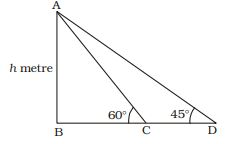Two consecutive kilometre stones ⇒ C and D
∠ADB = 45°; ∠ACB = 60°
CD = 1 km.
AB = height of plane = h metre
BC = x metre (let)
In ∆ABC,

 tan60° = AB BC

 ⇒ √3 = h x

⇒ h = √3x metre ..... (i)
In ∆ABD
 tan45° = AB BD

 ⇒ 1 = h x + 1

⇒ h = x + 1
 ⇒ h = h + 1 √3

[From equation (i)]
 ⇒ h - h = 1 √3

 ⇒ √3h - h = 1 √3

⇒ (√3 - 1)h = √3
 ⇒ h = √3 √3 - 1

 ⇒ h = √3(√3 + 1) (√3 - 1)(√3 + 1)

 ⇒ h = √3(√3 + 1) 2

 h = (3 + √3) metre 2

##### Correct Option: DTwo consecutive kilometre stones ⇒ C and D
∠ADB = 45°; ∠ACB = 60°
CD = 1 km.
AB = height of plane = h metre
BC = x metre (let)
In ∆ABC,

 tan60° = AB BC

 ⇒ √3 = h x

⇒ h = √3x metre ..... (i)
In ∆ABD
 tan45° = AB BD

 ⇒ 1 = h x + 1

⇒ h = x + 1
 ⇒ h = h + 1 √3

[From equation (i)]
 ⇒ h - h = 1 √3

 ⇒ √3h - h = 1 √3

⇒ (√3 - 1)h = √3
 ⇒ h = √3 √3 - 1

 ⇒ h = √3(√3 + 1) (√3 - 1)(√3 + 1)

 ⇒ h = √3(√3 + 1) 2

 h = (3 + √3) metre 2

1. Two men standing on same side of a pillar 75 metre high, observe the angles of elevation of the top of the pillar to be 30° and 60° respectively. The distance between two men is :
1. 100 √3 metre
2. 100 metre
3. 50 √3 metre
4. 25 √3 metre

1.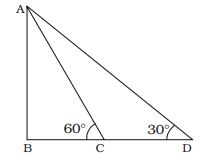AB = Height of pole = 75 metre
C and D ⇒ positions of persons
Let, BC = x metre, BD = y metre
∆ACB = 60°; ∆ADB = 30°
In ∆ABC,

 tan60° = AB BC

 ⇒ √3 = 75 x

 ⇒ x = 75 = 25 √3 metre √3

In ∆ABD
 tan30° = AB BD

 ⇒ 1 = 75 √3 y

⇒ y = 75 √3 metre
∴ CD = y – x = (75 √3 - 25 √3) metre CD = 50√3 metre

##### Correct Option: CAB = Height of pole = 75 metre
C and D ⇒ positions of persons
Let, BC = x metre, BD = y metre
∆ACB = 60°; ∆ADB = 30°
In ∆ABC,

 tan60° = AB BC

 ⇒ √3 = 75 x

 ⇒ x = 75 = 25 √3 metre √3

In ∆ABD
 tan30° = AB BD

 ⇒ 1 = 75 √3 y

⇒ y = 75 √3 metre
∴ CD = y – x = (75 √3 - 25 √3) metre CD = 50√3 metre

1. From a point P on a level ground, the angle of elevation to the top of the tower is 30°. If the tower is 100 metre high, the distance of point P from the foot of the tower is (Take √3 = 1.73)
1. 149 metre
2. 156 metre
3. 173 metre
4. 188 metre

1.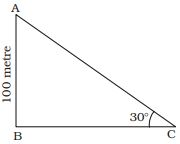Let, AB = Height of tower = 100 metre
∆ACB = 30°
In ∆ABC,

 tan30° = AB BC

 ⇒ 1 = 100 √3 BC

⇒ BC = 100√3 metre = (100 × 1.73) metre = 173 metre

##### Correct Option: CLet, AB = Height of tower = 100 metre
∆ACB = 30°
In ∆ABC,

 tan30° = AB BC

 ⇒ 1 = 100 √3 BC

⇒ BC = 100√3 metre = (100 × 1.73) metre = 173 metre

1. If the angle of elevation of the top of a pillar from the ground level is raised from 30° to 60°, the length of the shadow of a pillar of height 50 √3 will be decreased by
1. 60 metre
2. 75 metre
3. 100 metre
4. 50 metre

1.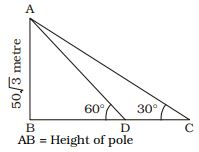AB = Height of pole = 50 √3 metre
BC = Length of shadow = x metre
When, ∠ACB = 30°
BD = Length of shadow = y metre
In ∆ABC,

 tan30° = AB BC

 ⇒ 1 = 50 √3 √3 x

⇒ x = 50√3 × √3 = 150 metre
In ∆ABD
 tan60° = AB BD

 ⇒ √3 = 50 √3 y

 ⇒ y = 50 √3 = 50 metre √3

∴ CD = x – y = 150 – 50 = 100 metre

##### Correct Option: CAB = Height of pole = 50 √3 metre
BC = Length of shadow = x metre
When, ∠ACB = 30°
BD = Length of shadow = y metre
In ∆ABC,

 tan30° = AB BC

 ⇒ 1 = 50 √3 √3 x

⇒ x = 50√3 × √3 = 150 metre
In ∆ABD
 tan60° = AB BD

 ⇒ √3 = 50 √3 y

 ⇒ y = 50 √3 = 50 metre √3

∴ CD = x – y = 150 – 50 = 100 metre

1. Find the angular elevation of the Sun when the shadow of a 15 metre long pole is (15 / √3) metre.
1. 45°
2. 60°
3. 30°
4. 90°

1.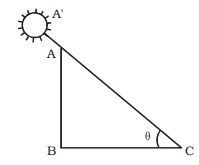A' ⇒ Position of sun
AB = Height of pole = 15 metre

 BC = Length of shadow = 15 metre √3

 ∴ tanθ = AB = 15 = √3 BC (15 / √3)

⇒ tanθ = tan60°
⇒ θ = 60°

##### Correct Option: BA' ⇒ Position of sun
AB = Height of pole = 15 metre

 BC = Length of shadow = 15 metre √3

 ∴ tanθ = AB = 15 = √3 BC (15 / √3)

⇒ tanθ = tan60°
⇒ θ = 60°